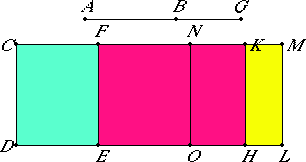# Proposition 97

The square on an apotome of a medial straight line applied to a rational straight line produces as breadth a first apotome.

Let AB be an apotome, and CD rational, and to CD let there be applied CE equal to the square on AB and producing CF as breadth.

I say that CF is a first apotome.

Let BG be the annex to AB. Then AG and GB are rational straight lines commensurable in square only.

X.73To CD apply CH, equal to the square on AG, and KL, equal to the square on BG.

II.7

Then the whole CL equals the sum of the squares on AG and GB, and, in these, CE equals the square on AB, therefore the remainder FL equals twice the rectangle AG by GB.

Bisect FM at the point N, and draw NO through N parallel to CD. Then each of the rectangles FO and LN equals the rectangle AG by GB.

Now, since the sum of the squares on AG and GB is rational, and DM equals the sum of the squares on AG and GB, therefore DM is rational.

X.20

And it is applied to the rational straight line CD producing CM as breadth, therefore CM is rational and commensurable in length with CD.

X.22

Again, since twice the rectangle AG by GB is medial, and FL equals twice the rectangle AG by GB, therefore FL is medial. And it is applied to the rational straight line CD producing FM as breadth, therefore FM is rational and incommensurable in length with CD.

Since the squares on AG and GB are rational, while twice the rectangle AG by GB is medial, therefore the sum of the squares on AG and GB is incommensurable with twice the rectangle AG by GB.

And CL equals the sum of the squares on AG and GB, and FL equals twice the rectangle AG by GB, therefore DM is incommensurable with FL.

But DM is to FL as CM is to FM, therefore CM is incommensurable in length with FM.

X.73

And both are rational, therefore CM and MF are rational straight lines commensurable in square only. Therefore CF is an apotome.

I say next that it is also a first apotome.

Since the rectangle AG by GB is a mean proportional between the squares on AG and GB, CH equals the square on AG, KL equals the square on BG, and NL equals the rectangle AG by GB, therefore NL is also a mean proportional between CH and KL. Therefore CH is to NL as NL is to KL.

But CH is to NL as CK is to NM, and NL is to KL as NM is to KM, therefore the rectangle CK by KM equals the square on NM, that is, the fourth part of the square on FM.

Since the square on AG is commensurable with the square on GB, therefore CH is also commensurable with KL.

But CH is to KL as CK is to KM, therefore CK is commensurable with KM.

X.17

Since CM and MF are two unequal straight lines, and to CM there has been applied the rectangle CK by KM equal to the fourth part of the square on FM and deficient by a square figure, while CK is commensurable with KM, therefore the square on CM is greater than the square on MF by the square on a straight line commensurable in length with CM.

X.Def.III.2

And CM is commensurable in length with the rational straight line CD set out, therefore CF is a first apotome.

Therefore, the square on an apotome of a medial straight line applied to a rational straight line produces as breadth a first apotome.

Q.E.D.

## Guide

This proposition is used in X.111. It is also used in proposition XIII.6.